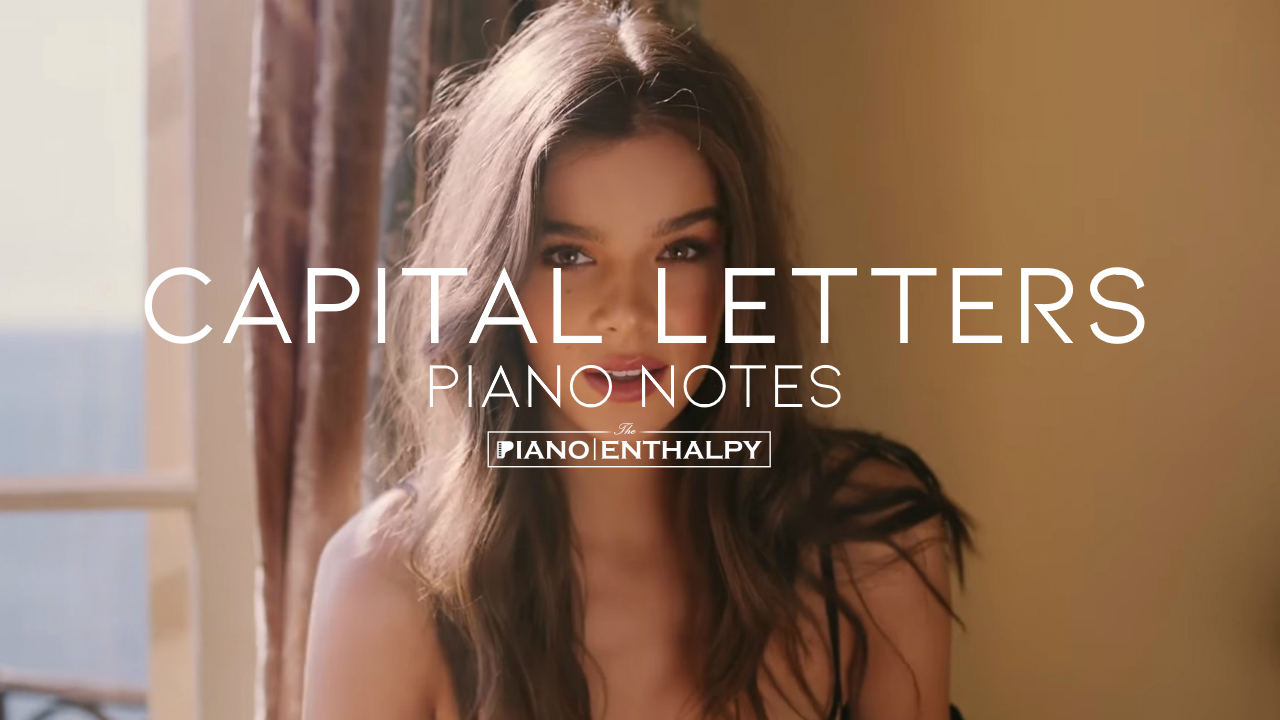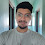# Hailee Steinfeld - Capital Letters | Piao NotesHailee Steinfeld - Capital Letters | Piao Notes

C+C+C+AC+E+
Never had a thing for fairytales
C+C+C+D+E+D+C+A
Not really a believer, oh-oh
AC+C+C+AC+E+, C+A
Small voice in the quiet
C+C+C+AC+E+
Guess I never dared to know myself
C+C+C+D+E+D+C+A
Can my heart beat quiet? No
AC+C+C+AC+E+, C+A

But then there was you (but then there was you)
AC+D+E+D+ (C+D+E+D+)
Yeah, then there was you
A,C+D+E+D+
Pull me out of the crowd
AAC+D+E+D+
You were telling the truth (you were telling the truth)
AAC+D+E+D+ (AAC+D+E+D+)
Yeah
C+
I got something to say now
AAC+D+E+D+ A
'Cause you tell me that there's
AAC+D+E+D+
No way I couldn't go
AAC+D+E+D+
Nothing I couldn't do
AAC+D+E+D+
Yeah
C+D++

I want to get louder
G+G+E+G+G+C++
I got to get louder
G+G+E+G+G+D++
We 'bout to go up baby, up we go
G+G+G+G+C++B+A+, B+A+E+
We 'bout to go up baby, up we go
G+G+G+G+C++B+A+, B+A+E+
We're blowing out speakers
G+G+E+G+G+C++
Our heart a little clearer
G+G+E+G+G+D++
We 'bout to go up baby, up we go
G+G+G+G+C++B+A+, B+A+E+
We 'bout to go up baby, up we go
G+G+G+G+C++B+A+, B+A+E+

For worst or for better
AC+D+E+D+C+
Gonna give it to you
AAC+D+E+D+
In capital letters
AC+D+E+D+C+

We put a crack in the shadows
C+C+C+AC+E+
And you tell me it's okay to be the light
C+C+C+D+E+D+C+A
And not to swim in the shallows, No, no
AC+C+C+AC+E+, C+A
And I wanna get drunk with you
C+C+A+G+E+E+D+D+
When we lie so still, but you're taking me places
C+C+A+G+E+, C+C+D+E+D+D+C+
Holding me onto you
C+D+E+D+C+A

And we don't care who's watching us, baby
But then there was you
AC+D+E+D+
(but then there was you)
(C+D+E+D+)
Yeah, then there was you
AC+D+E+D+
Pull me out of the crowd
AAC+D+E+D+
You were telling the truth
AAC+D+E+D+
(you were telling the truth)
AAC+D+E+D+
Yeah
C+A
I got something to say now
AAC+D+E+D+ A
'Cause you tell me that there's
AAC+D+E+D+
No way I couldn't go
AAC+D+E+D+
Nothing I couldn't do
AAC+D+E+D+
Yeah
C+D++

I want to get louder
G+G+E+G+G+C++
I got to get louder
G+G+E+G+G+D++
We 'bout to go up baby, up we go
G+G+G+G+C++B+A+, B+A+E+
We 'bout to go up baby, up we go
G+G+G+G+C++B+A+, B+A+E+
We're blowing out speakers
G+G+E+G+G+C++
Our heart a little clearer
G+G+E+G+G+D++
We 'bout to go up baby, up we go
G+G+G+G+C++B+A+, B+A+E+
We 'bout to go up baby, up we go
G+G+G+G+C++B+A+, B+A+E+

For worst or for better
AC+D+E+D+C+
Gonna give it to you
AAC+D+E+D+
In capital letters
AC+D+E+D+C+

Ohh..
C+BC+G+ D+E+E+F+E+D+

In capital letters
AC+D+E+D+C+
In capital–
AC+D+E+
Gonna give it to you
AAC+D+E+D+
Gonna give it to you
AAC+D+E+D+
Gonna give it to you
AAC+D+E+D+

I want to get louder
G+G+E+G+G+C++
I got to get louder
G+G+E+G+G+D++
We 'bout to go up baby, up we go
G+G+G+G+C++B+A+, B+A+E+
We 'bout to go up baby, up we go
G+G+G+G+C++B+A+, B+A+E+
We're blowing out speakers
G+G+E+G+G+C++
Our heart a little clearer
G+G+E+G+G+D++
We 'bout to go up baby, up we go
G+G+G+G+C++B+A+, B+A+E+
We 'bout to go up baby, up we go
G+G+G+G+C++B+A+, B+A+E+
[2 TIMES]

For worst or for better
AC+D+E+D+C+
Gonna give it to you
AAC+D+E+D+
In capital letters
AC+D+E+D+C+

1.